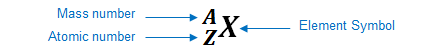# Problem: Identify the element represented by each of the following symbols and give the number of protons and neutrons in each.Give the number of protons and neutrons in 63152 X.

###### FREE Expert Solution

We’re being asked to determine the name of the element that has the following notation:

${}_{\mathbf{63}}{}^{\mathbf{152}}\mathbf{X}$

We are also asked to determine the number of protons and neutrons.

An element can be represented as:You can determine the identity of the element using its atomic number:

Atomic number (Z):

87% (197 ratings)###### Problem Details

Identify the element represented by each of the following symbols and give the number of protons and neutrons in each.

Give the number of protons and neutrons in 63152 X.